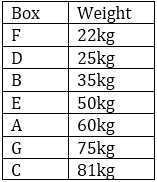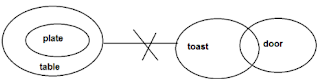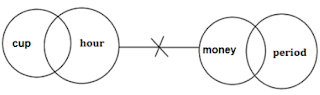# SBI PO 2019 Crash Course Reasoning Quiz: 4th June

### Reasoning Questions for SBI PO Prelims 2019

The SBI PO Prelims Exam is scheduled to be held in a few days and to ace your preparations Bankersadda is launching a crash course of 18 days to help you excel the exam. Here is a quiz on Reasoning Ability being provided by Adda247 for free to let you practice the best of latest pattern Reasoning Questions for SBI PO PRE Examination 2019-20. Video Solutions will also be provided for the same for free.

Directions (1-5): Study the following information carefully and answer the given questions:

Seven boxes are placed one above other, each box has different weight. All boxes are arranged in such a manner, lightest box is placed in top of the stack and the heaviest box is placed at bottom. One of the boxes is 75kg.

More than three boxes are placed in between box F and box G. Only two boxes are placed in between D and A which is 60kg. Three boxes are placed in between B and C. The third lightest box is 35kg. Box F is lighter than D. Box C is heavier than B. Weight of box E is double of box D. Box F is 13kg lighter than box B which is 10kg heavier than D. Difference between the weight of box A and C is 21kg.

Q1. What is the weight of the Second lightest Box?

28kg
25kg
30kg
21kg
20kg
Solution:

(i)- More than three boxes are placed in between box F and box G. The third lightest box is 35kg. Three boxes are placed in between B and C. Box C is heavier than B. That means box C is placed below Box B. Box F is lighter than D. There are three possibilities-(ii)- Only two boxes are placed in between D and A which is 60kg. By using this condition case-2 will be eliminated. Weight of box E is double of box D. By using this condition case-3 will be eliminated, and in case-1 position of box A and D has been fixed. Box F is 13kg lighter than box B which is 10kg heavier than D. Hence Box F is 22kg, D is 25kg and Box E is 50kg. Difference between the weight of box A and C is 21kg. The final arrangement is-Q2. Which of the following box is heavier than B but lighter than A?

C
D
E
F
G
Solution:

(i)- More than three boxes are placed in between box F and box G. The third lightest box is 35kg. Three boxes are placed in between B and C. Box C is heavier than B. That means box C is placed below Box B. Box F is lighter than D. There are three possibilities-(ii)- Only two boxes are placed in between D and A which is 60kg. By using this condition case-2 will be eliminated. Weight of box E is double of box D. By using this condition case-3 will be eliminated, and in case-1 position of box A and D has been fixed. Box F is 13kg lighter than box B which is 10kg heavier than D. Hence Box F is 22kg, D is 25kg and Box E is 50kg. Difference between the weight of box A and C is 21kg. The final arrangement is-Q3. How many boxes are lighter than D?

Four
Three
Two
One
None
Solution:

(i)- More than three boxes are placed in between box F and box G. The third lightest box is 35kg. Three boxes are placed in between B and C. Box C is heavier than B. That means box C is placed below Box B. Box F is lighter than D. There are three possibilities-(ii)- Only two boxes are placed in between D and A which is 60kg. By using this condition case-2 will be eliminated. Weight of box E is double of box D. By using this condition case-3 will be eliminated, and in case-1 position of box A and D has been fixed. Box F is 13kg lighter than box B which is 10kg heavier than D. Hence Box F is 22kg, D is 25kg and Box E is 50kg. Difference between the weight of box A and C is 21kg. The final arrangement is-Q4. What is the weight of the box which is placed exactly middle in the stack?

50kg
55kg
60kg
58kg
45kg
Solution:

(i)- More than three boxes are placed in between box F and box G. The third lightest box is 35kg. Three boxes are placed in between B and C. Box C is heavier than B. That means box C is placed below Box B. Box F is lighter than D. There are three possibilities-(ii)- Only two boxes are placed in between D and A which is 60kg. By using this condition case-2 will be eliminated. Weight of box E is double of box D. By using this condition case-3 will be eliminated, and in case-1 position of box A and D has been fixed. Box F is 13kg lighter than box B which is 10kg heavier than D. Hence Box F is 22kg, D is 25kg and Box E is 50kg. Difference between the weight of box A and C is 21kg. The final arrangement is-Q5. Which of the following box is placed at the bottom of the stack?

G
D
F
A
C
Solution:

(i)- More than three boxes are placed in between box F and box G. The third lightest box is 35kg. Three boxes are placed in between B and C. Box C is heavier than B. That means box C is placed below Box B. Box F is lighter than D. There are three possibilities-(ii)- Only two boxes are placed in between D and A which is 60kg. By using this condition case-2 will be eliminated. Weight of box E is double of box D. By using this condition case-3 will be eliminated, and in case-1 position of box A and D has been fixed. Box F is 13kg lighter than box B which is 10kg heavier than D. Hence Box F is 22kg, D is 25kg and Box E is 50kg. Difference between the weight of box A and C is 21kg. The final arrangement is-Directions (6-10): In each question below are given some statements followed by some conclusions numbered. You have to take the given statements to be true even if they seem to be at variance with commonly known facts. Read all the conclusions and then decide which of the given conclusions logically follows from the given statements, disregarding commonly known facts.

Q6. Statements:
Some scene are Old.
All old are shoes.
No shoe is a slipper.
Conclusions:
I. Some slippers are not scene.
II. All shoes being scene is a possibility.
III. At least some slippers are shoes.

Only I follows
Only I and II follow
Only II and III follow
All follow
None of these
Solution:Q7. Statements:
No holy is a bullet.
Some holy are foods.
Conclusions:
I. No radio is a holy.
II. Some foods are not bullets.
III. All foods are definitely not holy.

Only II and III follow
Only I and II follow
Only II follows
All I, II and III follow
None of these

Q8. Statements:
All plates are tables.
No table is a toast.
Some doors are toasts.
Conclusions:
I. some doors are definitely not tables.
II. All toasts are not tables.
III. No plate is a toast.

Only II and III follow
Only I and II follow
Only II follows
All I, II and III follow
None of these
Solution:Q9. Statements:
Some cups are hours.
No hour is a money.
Some periods are money.
Conclusions:
I. All periods are hours.
II. Some cups are not money.
III. Some moneys are not hours.

Only I and III follow
Only II follows
Only II and III follow
Only I and II follow
None of these
Solution:Q10. Statements:
No crop is water.
All waters are rains.
Some rains are huts.
Conclusions:
I. All crops being huts is a possibility.
II. Some waters are huts.
III. At least some huts are rains.

Only I and III follow
Only III follows
All I, II and III follow
Only II follows
None of these
Solution:Q11. The position of how many alphabets will remain unchanged if each of the alphabets in the word ‘SUBSCRIBE’ is arranged in alphabetical order from left to right?

None
One
Two
Three
More than Three
Solution:

Q12. What will come in place of question-mark (?) in the following series?
AZF     BYG     CXH       DWI      ?

ESJ
EUV
EVJ
DST
FRH
Solution:

Q13. Priya rank is 18th from the top and 6th from the bottom in the class. How many students are there in the class?

29
28
27
23
None of these
Solution:

Total number of students in the class= 18+6-1= 23

Q14.Among A, B, C, D and E, A is heavier than B but lighter than C. B is heavier than only E. C is not the heaviest. Who among them will be in the middle if they stand in the order of their weight?

A
C
B
D
E
Solution:

D>C>A>B>E

Q15. If 2 is multiply by all the even digits and 1 is Subtracted from all the odd digits in the number 1239475 then arrange all digits in descending order, then which of the following is second from the right end?

2
8
6
4
0
Solution:

Original number- 1239475
Obtain Number- 0428864

Descending order- 8864420

You may also like to Read: